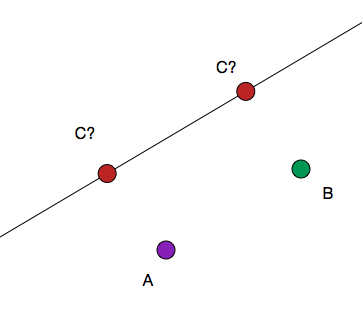# Warm up problem: Cartesian Right Triangle 2 (harder)

Geometry Level 4##### here is a link to the easier one

Given point A=(1,6) and B=(4,12) and line D=3x+7, a point C is placed on line D such that $\triangle ABC$ is a right triangle. If the sum of all possible x-coordinates of c can be represented by$\dfrac{p}{q}$. Find p+q.

Tip: the right angle doesn't necessarily occur at A or B.

×